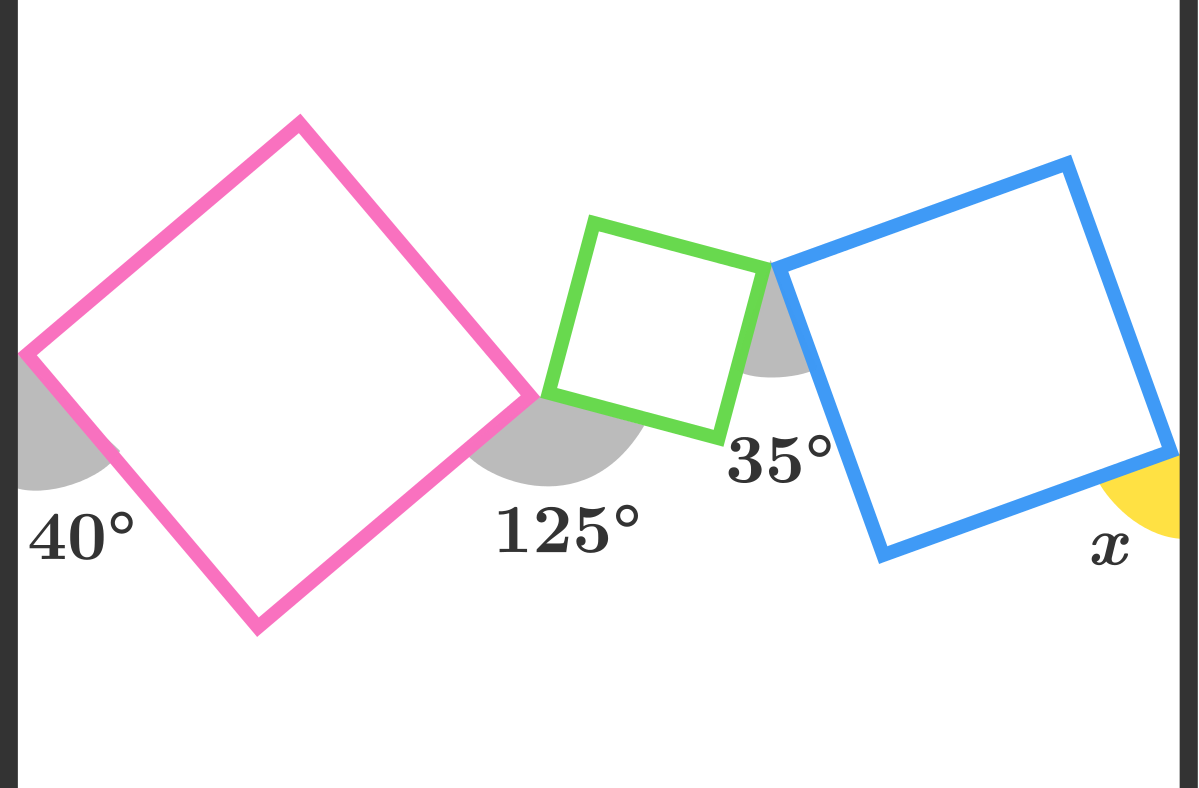# Three Squares in Two Parallel Lines

Geometry Level 1As shown in the diagram above, there lie 3 squares between 2 parallel lines such that each pair--(line, square) or (square, square)--just meet at a vertex. Find the measure of angle $x$ in degrees.

×

Problem Loading...

Note Loading...

Set Loading...